# 算法笔试题目总结（python传参**，yield)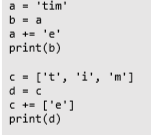考点：可变对象，不可变对象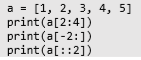考点：list切片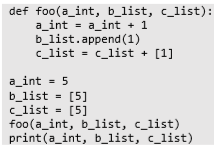考点：传参过程中可变对象，不可变对象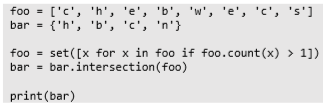考点：列表生成式，set，intersection()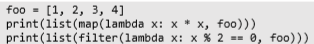考点：匿名函数lambda，filter()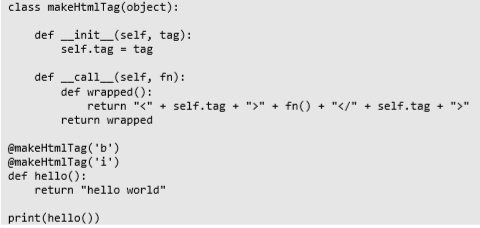考点：装饰器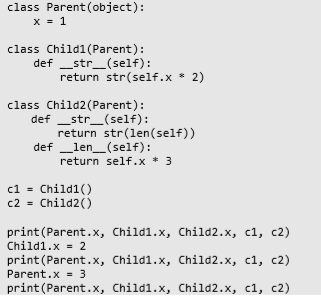考点：类与继承，实例对象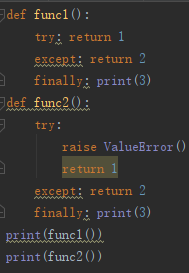考点：try-except-finally运行顺序

# 传参**，*

def foo(a, b='commit', *c, **d):
print(a, b, c, d)

foo(1, z='merge', b='clone', x=6, y=7)
foo(1, 2, 'push', 5, x='pull', y='chekcout')

# yield

def fibon(n):
a = b = 1
for i in range(n):
yield a
a, b = b, a + b

for item in fibon(5):
print(item)

# 列表生成式与dict

dic = {'a':1,'b':2}
dic2 = {key:dic[key]+1 for key in dic.keys()}
print(dic2) # 结果：{'a': 2, 'b': 3}

lis = ['a','b','c']
dic3 = {lis[i]:i for i in range(len(lis))}
print(dic3) # 结果：{'a': 0, 'b': 1, 'c': 2}